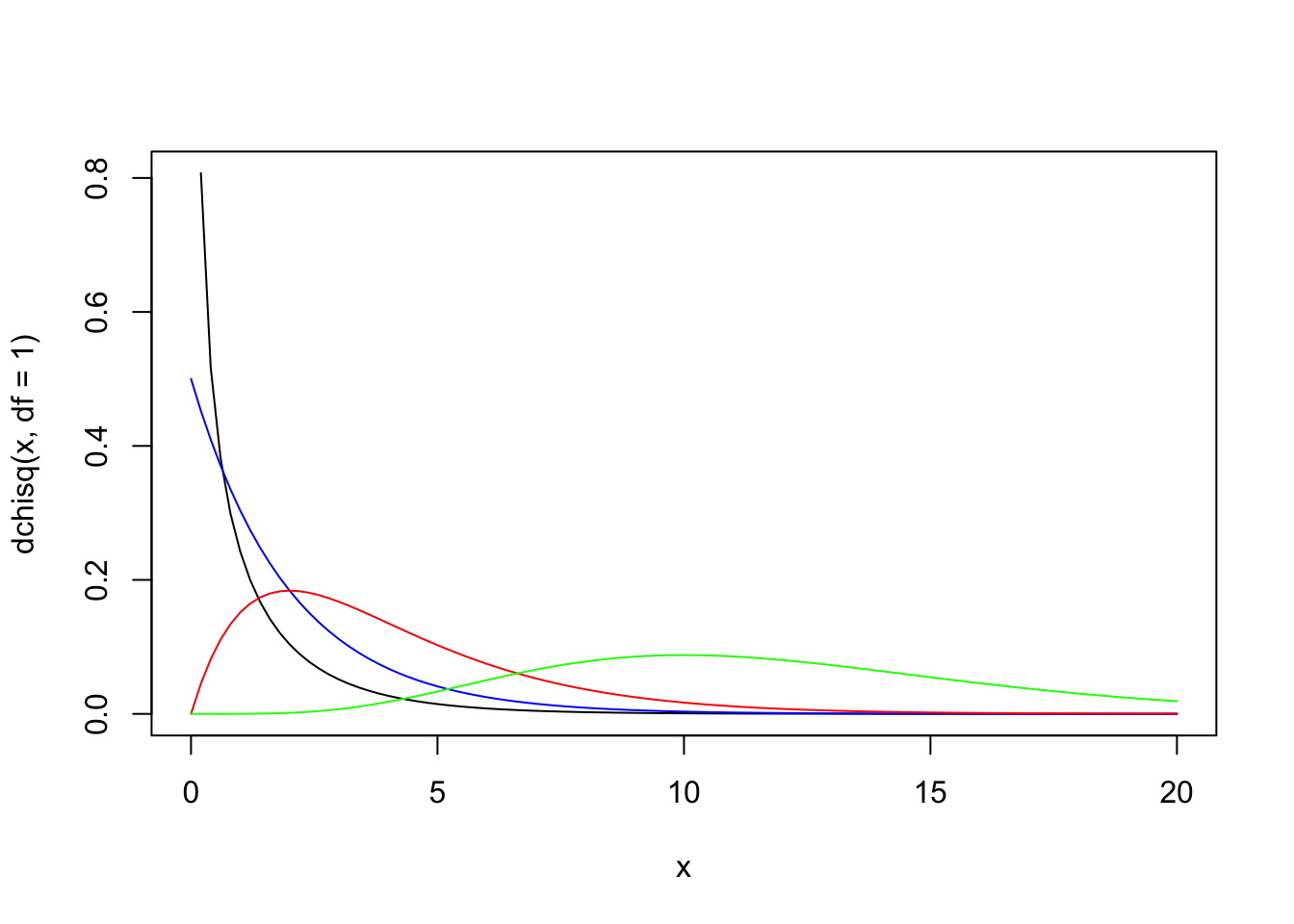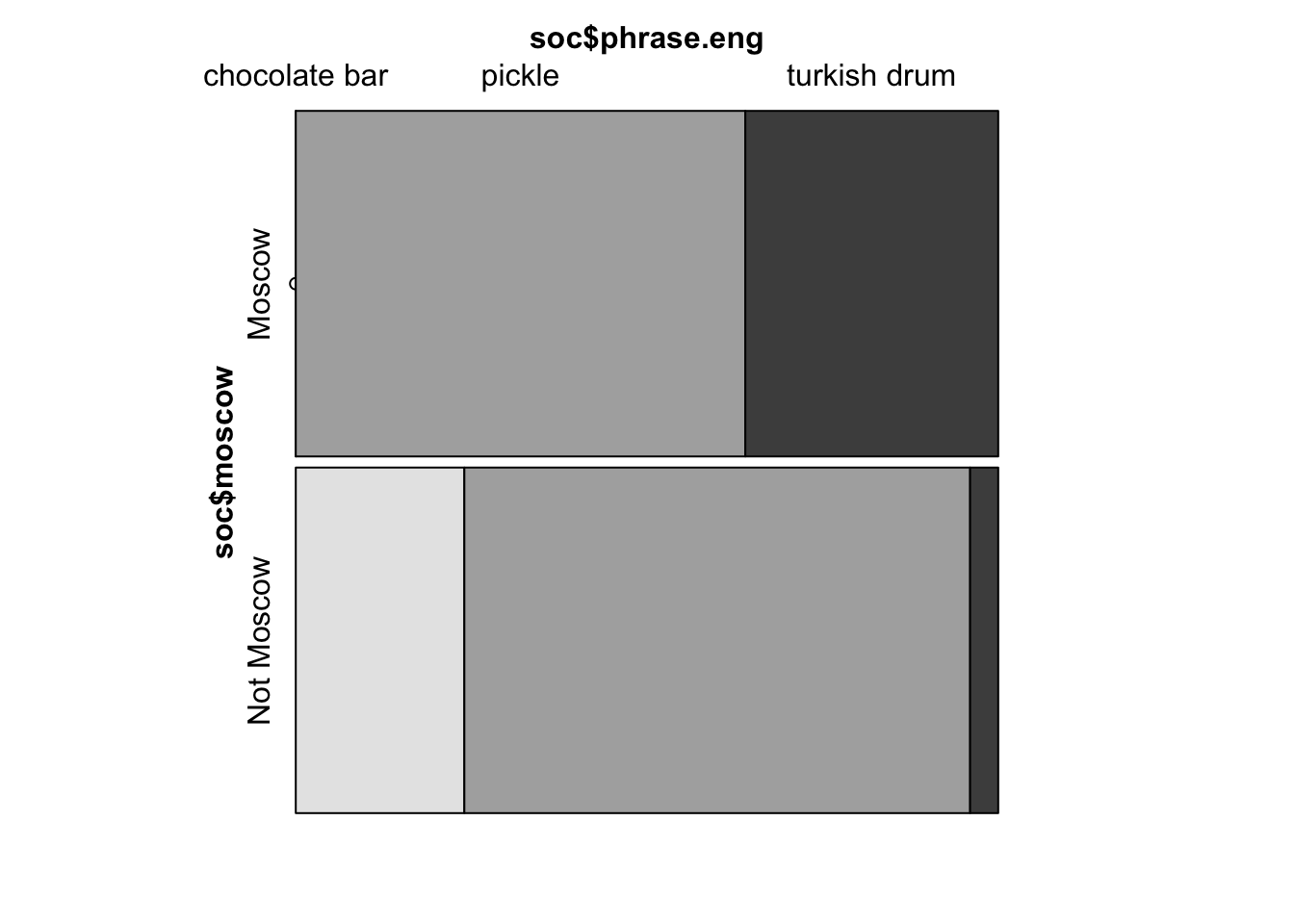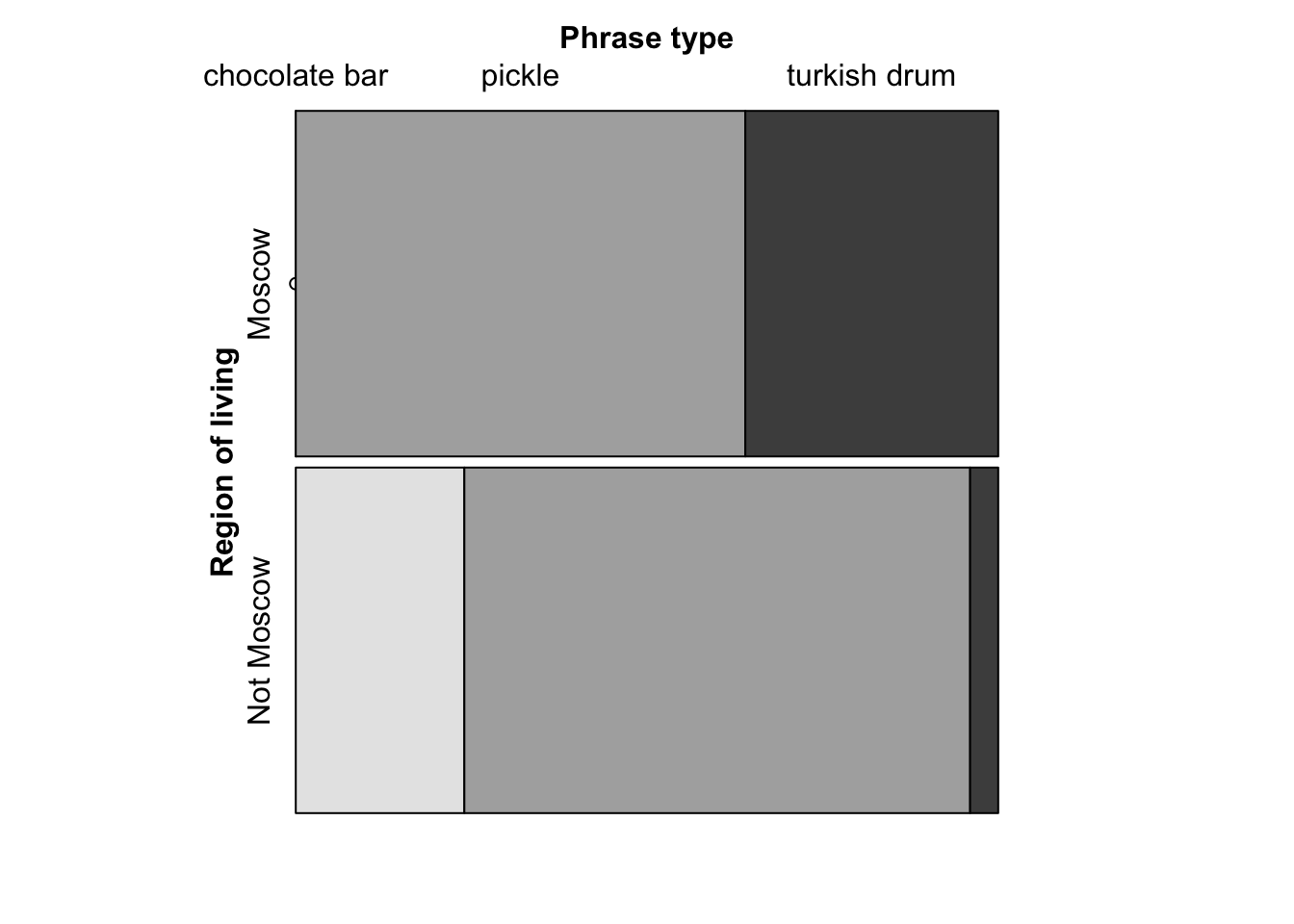### Part 1: contingency tables, chi-squared test and Fisher’s exact test

First, we will work with a data set that contains results of the following survey. 50 students of the Social Science faculty were asked how they continue the phrase “Жадина-говядина – …”, a phrase that is used in Russian to tease a greedy person. Variants of expression:

Variant 1

In Russian:

Жадина-говядина,

Солёный огурец,

На полу валяется,

Никто его не ест.

In English (word-by-word rendering approx.):

Piggy-wiggy

Is a pickle,

It lies on the floor,

And nobody eat it.

Variant 2

In Russian:

Жадина-говядина,

Турецкий барабан,

Кто на нём играет,

Тот рыжий таракан.

In English (word-by-word rendering approx.):

Piggy-wiggy

Is a Turkish drum,

Who plays on it,

Is a ginger cockroach.

Variant 3

In Russian:

Жадина-говядина,

Пустая шоколадина.

In English (word-by-word rendering approx.):

Piggy-wiggy

Is an empty chocolate bar.

Hence, we have three possible expressions to continue this teasing: using «солёный огурец» (pickle), «турецкий барабан» (Turkish drum) and «шоколадина» (chocolate bar).

Variables in a data set:

• id: respondent’s id (a numeric variable);
• region: region where a respondent lives (a factor variable);
• fdistrict: federal district where a respondent lives (a factor variable);
• sex: respondent’s sex (a factor variable, values: F for females, M for males);
• phrase: expression used in the phrase (a factor variable, values: солёный огурец, турецкий барабан, шоколадина);
• phrase.tr: a transliterated version of the expression used (a factor variable, values solenyj ogurec, tureckij baraban, šokoladina);
• phrase.eng: a word-by-word translation of the expression from Russian to English (a factor variable, values: pickle, turkish drum, chocolate bar).

Note: transliteration is done via the R library stringi.

soc <- read.csv("http://math-info.hse.ru/f/2018-19/pep/socling.csv")

Let us look at the summary of this data set:

summary(soc)
##        X               id                               region
##  Min.   : 1.00   Min.   : 1.00   Moscow                    :25
##  1st Qu.:15.25   1st Qu.:15.25   Moskovskaja oblast        : 4
##  Median :29.50   Median :29.50   Respublika Saha (Jakutija): 3
##  Mean   :29.30   Mean   :29.30   Samarskaja oblast         : 2
##  3rd Qu.:43.75   3rd Qu.:43.75   Volgogradskaja oblast     : 2
##  Max.   :57.00   Max.   :57.00   Altajskij kraj            : 1
##                                  (Other)                   :13
##         fdistrict  sex                 phrase              phrase.tr
##  Central     :32   F:34   соленый огурец  :34   šokoladina      : 6
##  Far Eastern : 4   M:16   турецкий барабан:10   solenyj ogurec  :34
##  Siberian    : 3          шоколадина      : 6   tureckij baraban:10
##  Volga       : 3
##  Northwestern: 2
##  (Other)     : 4
##          phrase.eng
##  chocolate bar: 6
##  pickle       :34
##  turkish drum :10
##
##
##
## 

As we have a lot of regions and a lot of federal districts, if we try to make a contingency table, we will have a lot of cells with very low frequencies or even with frequencies equal to zero. To avoid this, we can concentrate on the main distinction Moscow residents vs non-Moscow residents and aggregate our data correspondingly.

So, now our goal is to create a column moscow that contains values "Moscow" and "Not Moscow" and shows whether a respondent is from Moscow or not.

Task: Use tidyverse to add a variable moscow described above to our data set. Hint: in R there is the function ifelse() that works as follows:

v <- c('a', 'b', 'b', 'a')
ifelse(v == 'a', 1, 0)  # 1 if a, 0 is not a
##  1 0 0 1

Solution:

library(tidyverse)
soc <- soc %>% mutate(moscow = ifelse(region == "Moscow", "Moscow", "Not Moscow"))

Now let’s proceed to analysis.

Variables of interest: the expression used in teasing (phrase) and the region of living (whether Moscow or not, moscow).

Hypothesis of our small research: the type of expression used depends on the region of living.

First, we can create a contingency table:

tab <- table(soc$phrase, soc$moscow)
tab
##
##                    Moscow Not Moscow
##   соленый огурец       16         18
##   турецкий барабан      9          1
##   шоколадина            0          6

This table contains absolute frequencies. For instance, in our survey 16 people from Moscow used соленый огурец while teasing, one person not from Moscow used турецкий барабан while teasing, no people from Moscow used шоколадина, and so on.

We can create a table with relative frequencies:

# a contingency table tab is inside
prop.table(tab)
##
##                    Moscow Not Moscow
##   соленый огурец     0.32       0.36
##   турецкий барабан   0.18       0.02
##   шоколадина         0.00       0.12

Or even with percentages:

prop.table(tab) * 100 # in %
##
##                    Moscow Not Moscow
##   соленый огурец       32         36
##   турецкий барабан     18          2
##   шоколадина            0         12

Question. Judging by this table, can you say that the type of expression used depends on the region of living?

Answer. Although we can see the difference between frequencies in a table, still formal testing is needed.

Statistical hypotheses:

$$H_0:$$ The type of expression used in teasing does not depend on the region of living.

$$H_1:$$ The type of expression used in teasing depends on the region of living.

As our variables of interest are categorical (nominal or qualitative), we should use a chi-squared test.

Let’s perform a chi-squared test in R:

# variables of interest are in brackets,
# not a contingency table itself
chisq.test(soc$phrase, soc$moscow)
## Warning in chisq.test(soc$phrase, soc$moscow): Chi-squared approximation
## may be incorrect
##
##  Pearson's Chi-squared test
##
## data:  soc$phrase and soc$moscow
## X-squared = 12.518, df = 2, p-value = 0.001913

Statistical interpretation. P-value is 0.002 that is less than 0.05, so at the 5% significance level we have grounds to reject the null hypothesis about the independence of two factor variables.

Substantial interpretation. The type of expression used in teasing depends on the region of living. People who live in Moscow and people who do not live in Moscow use different versions of teasing.

As we can see, while performing a chi-squared test, we got a warning that chi-squared approximation may be incorrect. This usually happens when there are expected frequencies less than 5. In some statistical packages the percentage of such cells is also considered: they return a warning if in a contingency table is greater than 5% or 10%.

So as to get more exact results, we can use an exact Fisher’s test (the null hypothesis is the same).

fisher.test(soc$phrase, soc$moscow)
##
##  Fisher's Exact Test for Count Data
##
## data:  soc$phrase and soc$moscow
## p-value = 0.001481
## alternative hypothesis: two.sided

In our case the p-value obtained in a Fisher’s test is slightly different from the p-value from a chi-squared test. So, even if we consider a more precise test, our conclusions will not change.

Now let’s investigate the output of the chi-squared test in more detail. First, save results into a variable and call it res:

res <- chisq.test(soc$phrase, soc$moscow)
## Warning in chisq.test(soc$phrase, soc$moscow): Chi-squared approximation
## may be incorrect

Look at the expected frequencies: frequencies that are expected provided that our null hypothesis holds true, so if the expression used and the region of living are independent:

res$expected ## soc$moscow
## soc$phrase Moscow Not Moscow ## соленый огурец 17 17 ## турецкий барабан 5 5 ## шоколадина 3 3 Look at the observed frequencies (the same as we have got in the original contingency table): res$observed
##                   soc$moscow ## soc$phrase         Moscow Not Moscow
##   соленый огурец       16         18
##   турецкий барабан      9          1
##   шоколадина            0          6

In the R output we have seen that the chi-squared value equals 12.518. Let’s recall the formula from the lecture and try to get this value using frequencies from R.

The formula:

$\chi^2 = \sum\limits_{i=1}^n\frac{(observed - expected)^2}{expected}$

So, we for each cell in a contingency table we should subtract an expected frequency from the observed one, square the result and divide it by the expected frequency. And then, sum up the results for every cell. Let’s do it in R:

# by hand - for the sake of clarity
(18 - 17)^2 / 17 + (16 - 17)^2 / 17 + (1 - 5)^2 / 5 + (9 - 5)^2 / 5 + (6 - 3)^2 / 3 + (0 - 3)^2 / 3 
##  12.51765
# using R tables - more convenient
sum((res$expected - res$observed) ** 2 / res$expected) ##  12.51765 At the seminar several questions about a chi-squared distribution were raised. First, how this distribution looks like, and, second, what is df in the R output. The df in the output stands for degrees of freedom. It is a parameter of a chi-squared distribution that is responsible for its shape. The larger is the number of degrees of freedom, the flatter a density graph of the distribution is. Let’s plot chi-squared density functions for different df (recall: you can consider a density function as a smoothed line for a histogram): curve(dchisq(x, df = 1), xlim = c(0, 20)) curve(dchisq(x, df = 2), xlim = c(0, 20), col = "blue", add = TRUE) curve(dchisq(x, df = 4), xlim = c(0, 20), col = "red", add = TRUE) curve(dchisq(x, df = 12), xlim = c(0, 20), col = "green", add = TRUE)Two more questions are left: • What is df? • Why in our case df = 2? The informal answer to the first question is the following. The degrees of freedom is the number of independent pieces of information that we need to estimate a parameter of a distribution. Consider an example. We want to fill in a contigency table where marginal frequencies (row sums and column sums) are known. Males Females Total Use feminitives ? ? 40 Don’t use feminitives ? ? 40 Total 30 50 80 How many cells should we know to do this? One cell is enough! If we know that there are 10 males who use feminitives, we can freely compute frequencies in other cells using row sums and column sums: Males Females Total Use feminitives 10 ? 40 Don’t use feminitives ? ? 40 Total 30 50 80 Calculate: Males Females Total Use feminitives 10 40 - 10 = 30 40 Don’t use feminitives 30 - 10 = 20 50 - 30 = 20 40 Total 30 50 80 So, degrees of freedom here equals to one, df = 1. The second question is easy: df we get in the output for a chi-squared test is computed in the following way: $\text{df} = (r-1)\cdot (c-1),$ where $$r$$ is a number of rows in a contingency table for our variables of interest and $$c$$ is a number of columns in this table. That is why in the output above we got 2: $$\text{df} = (3-1)\cdot(2-1) = 2$$. ### Part 2: Visualisation of contingency tables Now let us visualise a contingency table via vcd library (from visualising categorical data). Install this library first: install.packages("vcd") Create a mosaic plot. By x-axis we will place frequencies for the variable phrase.eng (labels in English are shorter, so we use them), by y-axis we will place frequencies for the variable moscow. library(vcd) mosaic(soc$phrase.eng ~ soc$moscow)From this graph we can see that in Moscow people use солёный огурец (pickle) and турецкий барабан (turkish drum) while teasing and people not from Moscow rarely use турецкий барабан (turkish drum), but often say phrases with солёный огурец (pickle) and шоколадина (chocolate bar). Now we will not focus on styling, later we will see how to create a mosaic plot via ggplot2, however, let us name axes in a more pretty way: mosaic(data = soc, phrase.eng ~ moscow, set_varnames = list(phrase.eng = "Phrase type", moscow = "Region of living"))### Part 3: chi-squared test and effect size Now you are suggested to perform a chi-squared on your own using a different data set. phono <- read.csv("https://raw.githubusercontent.com/LingData2019/LingData/master/data/elision.csv") This data set contains data for the research [V.Sveshnikova] on the elision of the labio-dental [v] in cases when it is followed by another labio-dental consonant (one context, -ствов, like in бесчинствовать) and in cases when there is no labio-dental sound after [v] (two contexts, like in бесчинствую, бесчинство). Variables: • v.elision — [v] or not; • group — a group of words, first (like бесчинствовать), second (like бесчинствую), third (like бесчинство); • word — a root of a word; • position — position in (strong and weak). Task: How do you feel: does the elision of [v] depends on the context, namely on the presence of another labio-dental consonant afterwards? Formulate a substantial linguistic hypothesis, then formulate statistical $$H_0$$ and $$H_1$$ and test the null hypothesis. Interpret the results obtained. Solution: Linguistic hypothesis: the elision of [v] in Russian depends on the context, i.e. on the presence of a labio-dental consonant afterwards. Statistical hypotheses: $$H_0:$$ the elision of [v] does not depend on the context (v.elision and group are independent). $$H_1:$$ the elision of [v] depends on the context (v.elision and group are not independent, they are associated). Formal testing: A contingency table: tab2 <- table(phono$v.elision, phono$group) tab2 ## ## first second third ## no 2 32 92 ## yes 170 151 144 A chi-squared test: chisq.test(phono$v.elision, phono$group) ## ## Pearson's Chi-squared test ## ## data: phono$v.elision and phono\$group
## X-squared = 87.158, df = 2, p-value < 2.2e-16

Interpretation. At the 5% significance level we can reject the null hypothesis about the independence of two categorical variables. The elision of [v] depends on the context, on the presence of a labio-dental consonant afterwards.

Now let’s briefly discuss a way to measure an effect size you mentioned at the lecture, a Cramer’s V. A statistical significance that we evaluate based on the p-value does not necessarily mean real significance in practice. For example, the association between two variables can be statistically significant, but the strength of this association can be very small. To decide whether the relationship between two categorical variables is important, ‘really’ significant, a Cramer’s V is used. This measure is calculated as follows:

$\text{Cramer's V} = \sqrt{\frac{\chi^2 / n }{\min\{r-1, c-1\}}},$

where $$\chi^2$$ is the observed value of the statistics (X-squared in the R output), $$n$$ is a total number of observations, $$r$$ is a number of rows in a contingency table, $$c$$ is a number of columns in a contingency table.

To get this value in R we will need a library lsr:

install.packages("lsr")

Then, simply pass a contingency table to the function cramersV():

library(lsr)
cramersV(tab2)
##  0.3840262

How to interpret this value? Generally, a Cramer’s V takes values from 0 to 1 where 1 corresponds to the strongest association (two variables are identical). Here it is 0.38, so we can conclude that although the elision of [v] depends on the presence of a labio-dental consonant afterwards, this association is not very strong.